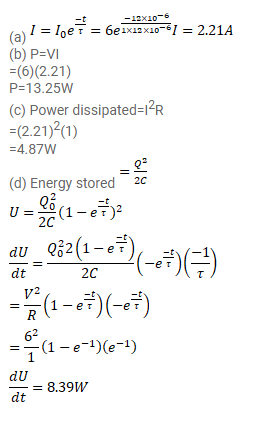# A capacitor of capacitance 12.0μF is connected to

Question:

A capacitor of capacitance $12.0 \mu \mathrm{F}$ is connected to a battery of emf $6.00 \mathrm{~V}$ and internal resistance1.00 $\Omega$ through resistance less leads. $12.0 \mu \mathrm{s}$ after the connections are made, what will be

(a) The current in the circuit,

(b) The power delivered by the battery,

(c) The power dissipated in heat and

(d) The rate at which the energy stored in the capacitor is increasing.

Solution: